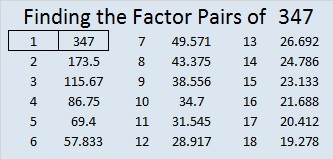# Prime Number Tests for 347

• 347 is a prime number.
• Prime factorization: 347 is prime.
• The exponent of prime number 347 is 1. Adding 1 to that exponent we get (1 + 1) = 2. Therefore 347 has exactly 2 factors.
• Factors of 347: 1, 347
• Factor pairs: 347 = 1 x 347
• 347 has no square factors that allow its square root to be simplified. √347 ≈ 18.628How do we know that 347 is a prime number? If 347 were not a prime number, then it would be divisible by at least one prime number less than or equal to √347 ≈ 18.628. Since 347 cannot be divided evenly by 2, 3, 5, 7, 11, 13, or 17, we know that 347 is a prime number.

Is there a quicker way to tell that 347 is a prime number? Not exactly…There is a quick test that will tell you if 347 is VERY LIKELY prime.

Quick Prime or Composite Number Test: You can use as few as TEN calculator keystrokes to test if 347 is VERY LIKELY prime: What is the remainder when 2³⁴⁷ is divided by 347? To find out type “(2)(xʸ)(3)(4)(7)(Mod)(3)(4)(7)” followed by the equal sign into your computer’s calculator. This is how the calculator should look before you hit the equal sign:2^347 mod (347) = 2, the same as the base we typed in. That means 347 is VERY LIKELY prime. This tests works for all prime numbers, but sometimes it gives a false positive for a relatively few (but infinite number) of composite numbers. (341 is the smallest of these numbers, and I wrote about it here.)

2 isn’t the only prime number that can be used as the base in this quick test. You can verify the following also on your computer’s calculator:

• 3^347 mod (347) = 3
• 5^347 mod (347) = 5
• 7^347 mod (347) = 7
• 11^347 mod (347) = 11. We can practically go on forever using prime number after prime number….until
• 337^347 mod (347) = 337, the largest prime number less than 347.

As long as we use a prime less than 347 as the base and 347 as the exponent, we will always get that prime as the remainder. Still even though 347 passes ALL those prime number tests, we can only conclude that 347 is VERY LIKELY prime. Doing all those calculations is more work than simply dividing 347 by all the prime numbers less than or equal to its square root and getting a remainder every single time.

Tomcircle, a fellow blogger, shared a video of a new-prime-number-test that works for prime numbers and NEVER gives a false positive.

While this test works every time in theory, it can be quite a nightmare in practice. It involves putting the possible prime number into a particular expression, expanding the expression, calculating each coefficient, and verifying that all those coefficients are divisible by this possible prime number. In the case of the relatively small number 347, there would be 173 different coefficients. Each of those coefficients are the numbers in the 347th row of Pascal’s triangle, and too many of them are usually expressed in scientific notation. Dividing each of them by 347 to verify there is no remainder is far more work than most people would want to do. In fact, dividing 347 by all the integers less than 347 would actually be less work!

The Sieve of Eratosthenes was how the ancient Greeks found prime numbers. It takes a little longer than dividing the possible prime number by all of the primes less than or equal to its square root, but it finds many primes at the same time. Solvemymath shares some interesting facts about it.

Prime numbers are intriguing, and 347 is one of them!